français | español | polski | 日本語 |

Pokemon math blaster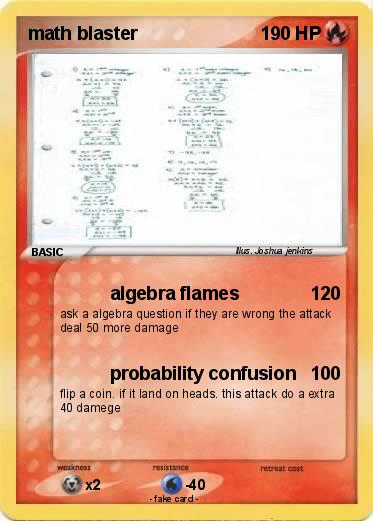Language Card : english

Creation Date : 21 November 2013

### Pokemon Passport

Name : math blaster

Serie : 1st generation

Type : Fire

Attack 1 : algebra flames
ask a algebra question if they are wrong the attack deal 50 more damage

Attack 2 : probability confusion
flip a coin. if it land on heads. this attack do a extra 40 damege

Illustrator : Joshua jenkins

### Vote for this card

(1 vote)

Related cards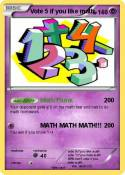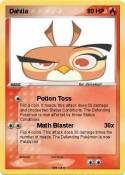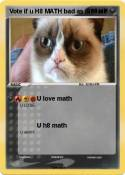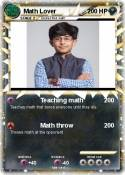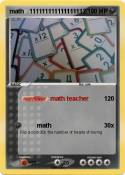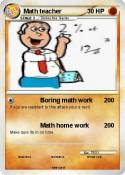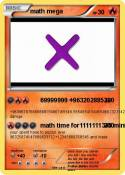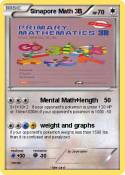xtra math teacher | JiJi ST math | The Great Math Wolf | Foxeh the math Fox | Khan academy | Vote 5 if you hate math | Math Mann | Ratey the Math Cat | xtra math guy | xtra math guy | I Can Do Math Everyday! | MATH | Math Teacher | MATh | math team | Math Book | Math knight | Brice | Math | Math Teacher | Math :( | math 100 x | me in math | Math man | Daniel EX | Albert Einstein | MATH | MATH | Math Gunner | Nerd | math | Math Homework | Cool Math | math doesn't lie | Tushar | Math 24 | Math Knight | Math Man | Math | Boring Math Teacher | Math | Mrs Ferriola | 1+1 | Ratey hte math cat | St Math | Mrs Baker | baldi | Math | math | math | St math | math | Math | Math | prodigy | Math | Math | more math | math | Asian guy | Asian guy | School | Math | prodigy guy | extra math guy | xtramath guy | xtramath guy | Inferno blaster | Gaster Blaster sans | Math Quiz